# NCERT Solutions for Class 9 Maths Exercise 2.1## myCBSEguide App

CBSE, NCERT, JEE Main, NEET-UG, NDA, Exam Papers, Question Bank, NCERT Solutions, Exemplars, Revision Notes, Free Videos, MCQ Tests & more.

NCERT solutions for Class 9 Maths Polynomials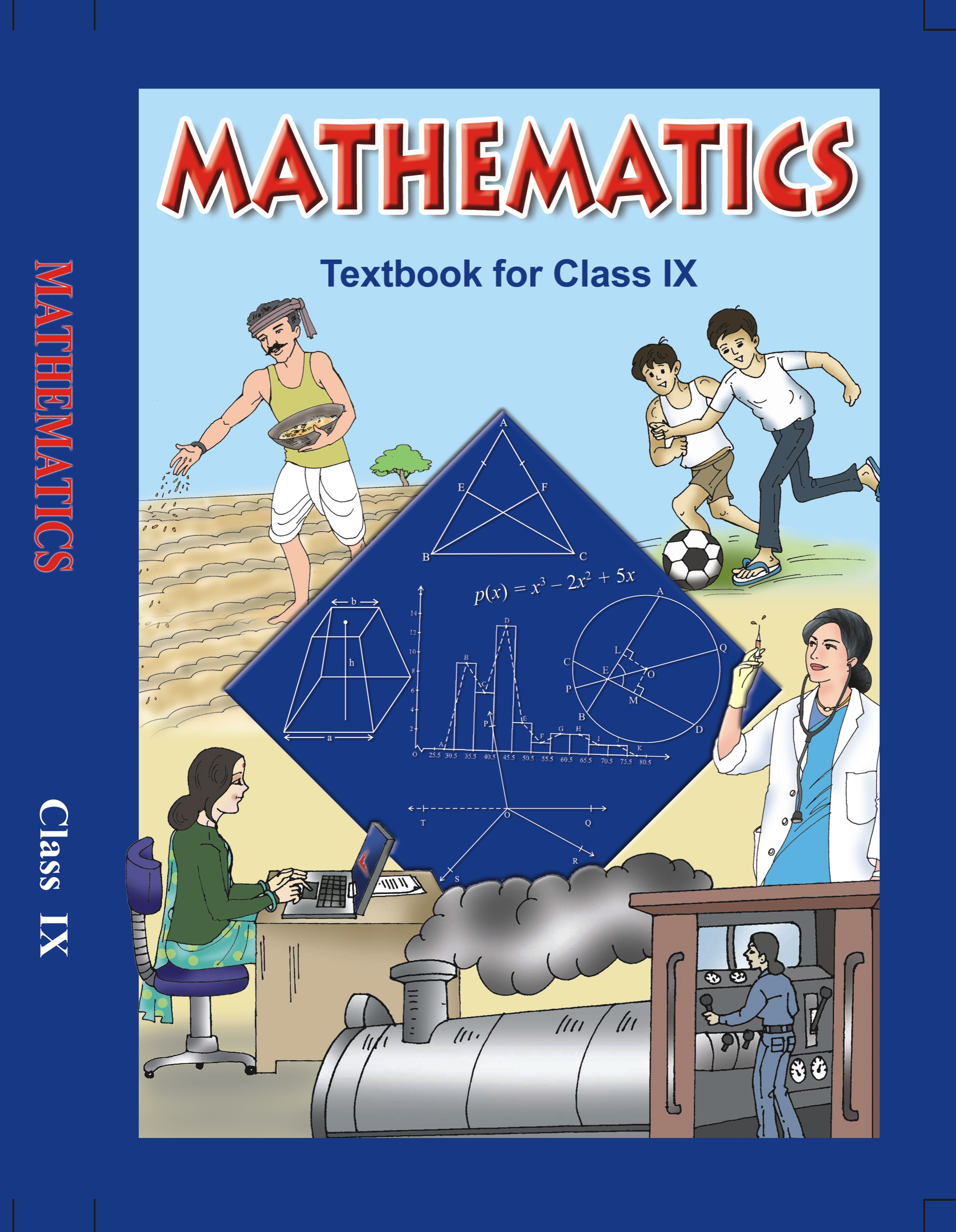## NCERT Solutions for Class 9 Maths Polynomials

###### 1. Which of the following expressions are polynomials in one variable and which are not? State reasons for your answer.

(i)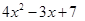(ii)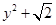(iii)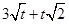(iv)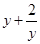(v)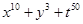Ans. (i)We can observe that in the polynomial, we have x as the only variable and the powers of x in each term are a whole number.

Therefore, we conclude thatis a polynomial in one variable.

(ii)We can observe that in the polynomial, we have y as the only variable and the powers of y in each term are a whole number.

Therefore, we conclude thatis a polynomial in one variable.

(iii)We can observe that in the polynomial, we have t as the only variable and the powers of t in each term are not a whole number.

Therefore, we conclude thatis not a polynomial in one variable.

(iv)We can observe that in the polynomial, we have y as the only variable and the powers of y in each term are not a whole number.

Therefore, we conclude thatis not a polynomial in one variable.

(v)We can observe that in the polynomial, we have x, y and t as the variables and the powers of x, y and t in each term is a whole number.

Therefore, we conclude thatis a polynomial but not a polynomial in one variable.

NCERT Solutions for Class 9 Maths Exercise 2.1

###### 2. Write the coefficients of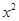in each of the following:

(i)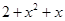(ii)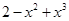(iii)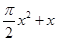(iv)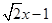Ans. (i)The coefficient ofin the polynomialis 1.

(ii)The coefficient ofin the polynomialis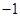.

(iii)The coefficient ofin the polynomialis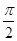.

(iv)The coefficient ofin the polynomialis 0.

NCERT Solutions for Class 9 Maths Exercise 2.1

###### 3. Give one example each of a binomial of degree 35, and of a monomial of degree 100.

Ans. The binomial of degree 35 can be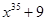.

The binomial of degree 100 can be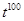.

NCERT Solutions for Class 9 Maths Exercise 2.1

###### 4. Write the degree of each of the following polynomials:

(i)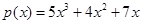(ii)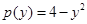(iii)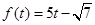(iv)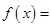3

Ans. (i)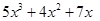We know that the degree of a polynomial is the highest power of the variable in the polynomial.

We can observe that in the polynomial, the highest power of the variable x is 3.

Therefore, we conclude that the degree of the polynomialis 3.

(ii)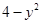We know that the degree of a polynomial is the highest power of the variable in the polynomial.

We can observe that in the polynomial, the highest power of the variable y is 2.

Therefore, we conclude that the degree of the polynomialis 2.

(iii)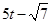We know that the degree of a polynomial is the highest power of the variable in the polynomial.

We observe that in the polynomial, the highest power of the variable t is 1.

Therefore, we conclude that the degree of the polynomialis 1.

(iv)3

We know that the degree of a polynomial is the highest power of the variable in the polynomial.

We can observe that in the polynomial 3, the highest power of the assumed variable x is 0.

Therefore, we conclude that the degree of the polynomial 3 is 0.

NCERT Solutions for Class 9 Maths Exercise 2.1

###### 5. Classify the following as linear, quadratic and cubic polynomials:

(i)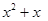(ii)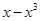(iii)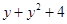(iv)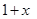(v) 3t

(vi)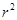(vii)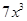Ans. (i)We can observe that the degree of the polynomialis 2.

Therefore, we can conclude that the polynomialis a quadratic polynomial.

(ii)We can observe that the degree of the polynomialis 3.

Therefore, we can conclude that the polynomialis a cubic polynomial.

(iii)We can observe that the degree of the polynomialis 2.

Therefore, the polynomialis a quadratic polynomial.

(iv)We can observe that the degree of the polynomial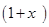is 1.

Therefore, we can conclude that the polynomialis a linear polynomial.

(v)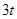We can observe that the degree of the polynomial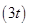is 1.

Therefore, we can conclude that the polynomialis a linear polynomial.

(vi)We can observe that the degree of the polynomialis 2.

Therefore, we can conclude that the polynomialis a quadratic polynomial.

(vii)We can observe that the degree of the polynomialis 3.

Therefore, we can conclude that the polynomialis a cubic polynomial.

## NCERT Solutions for Class 9 Maths Exercise 2.1

NCERT Solutions Class 9 Maths PDF (Download) Free from myCBSEguide app and myCBSEguide website. Ncert solution class 9 Maths includes text book solutions from Mathematics Book. NCERT Solutions for CBSE Class 9 Maths have total 15 chapters. 9 Maths NCERT Solutions in PDF for free Download on our website. Ncert Maths class 9 solutions PDF and Maths ncert class 9 PDF solutions with latest modifications and as per the latest CBSE syllabus are only available in myCBSEguide.

## CBSE app for Class 9

To download NCERT Solutions for Class 9 Maths, Computer Science, Home Science,Hindi ,English, Social Science do check myCBSEguide app or website. myCBSEguide provides sample papers with solution, test papers for chapter-wise practice, NCERT solutions, NCERT Exemplar solutions, quick revision notes for ready reference, CBSE guess papers and CBSE important question papers. Sample Paper all are made available through the best app for CBSE students and myCBSEguide website.1. Very good

2. Kk